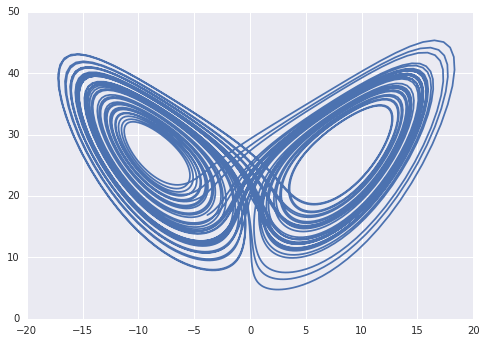# W3cubDocs

/TensorFlow Python

# Integrate (contrib)

Integration and ODE solvers for TensorFlow.

## Example: Lorenz attractor

We can use `odeint` to solve the Lorentz system of ordinary differential equations, a prototypical example of chaotic dynamics:

```rho = 28.0
sigma = 10.0
beta = 8.0/3.0

def lorenz_equation(state, t):
x, y, z = tf.unstack(state)
dx = sigma * (y - x)
dy = x * (rho - z) - y
dz = x * y - beta * z
return tf.stack([dx, dy, dz])

init_state = tf.constant([0, 2, 20], dtype=tf.float64)
t = np.linspace(0, 50, num=5000)
tensor_state, tensor_info = tf.contrib.integrate.odeint(
lorenz_equation, init_state, t, full_output=True)

sess = tf.Session()
state, info = sess.run([tensor_state, tensor_info])
x, y, z = state.T
plt.plot(x, z)
```